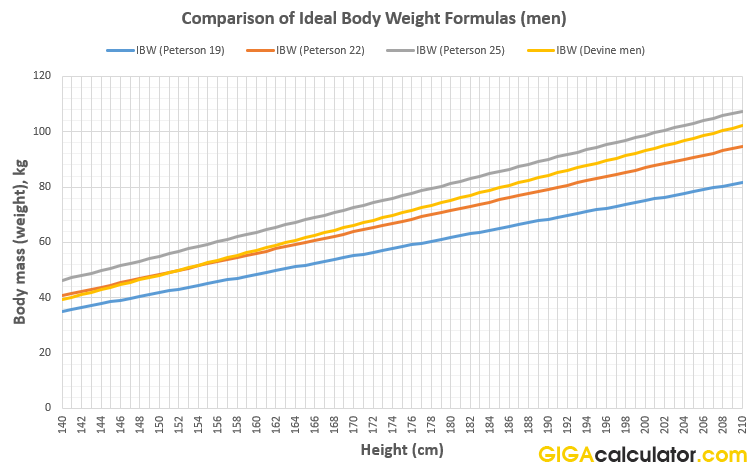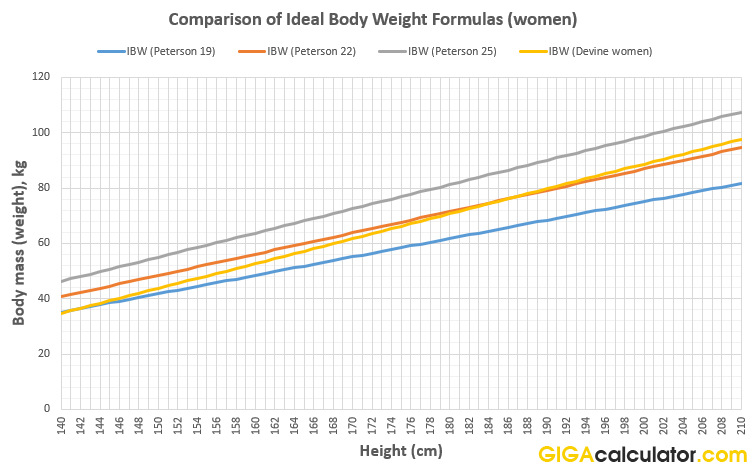# Ideal Weight Calculator

Use this calculator to estimate your ideal weight based on your age and height, using the novel Peterson equation for ideal body weight, but also providing the result from the popular Devine IBW formula for reference.

ft
in

### Calculation results

153.9 lbs Ideal Weight (Peterson) Ideal Weight (Devine)
Share calculator:

Embed this tool:
get code

## How to calculate ideal weight?

There are multiple formulas for calculating ideal body weight, with the first being invented as early as 1871 by Dr. Pierre Paul Broca, a French surgeon. Much later, in 1974 Dr. B.J. Devine published his famous equation which basically converted a simple rule based on the Broca ideal body weight formula from pounds to kilograms. It is the one used in many IBW calculators, but in our ideal body weight calculator we chose a different approach and we will explain why (we also show the Devine formula result, for reference).

The major issue with the Devine formula is that it is not scientific. Unlike other health formulas, it was not derived from any kind of systematic research, regression analysis, etc. but was based on estimates from Devine's mentor . Validation of the formula's results versus actual lean body mass and average body mass revealed that the formula's results are skewed at both ends of the spectrum - they underestimate body weight at shorter heights and overestimate it for persons of higher stature. The issue was not really solved by the formulas provided by the works of Robinson et al. and Miller et al. in 1983. These issues make IBW equations as a whole incompatible with BMI and combined with the fact that they only prescribe a single value makes them not very suitable, even for their intended purpose. BMI ranges for healthy body weight are often used instead.

In attempting to address these shortcomings of the Devine and later formulas, Peterson at al.  produced in 2016 a novel body weight equation that is more accurate than past IBW equations and is generalizable to any target BMI and height. Its accuracy was evaluated through theoretical and empirical means (the latter by applying it to historical data from adults, participating in the 1999-2006 NHANES). The maximum percent error across the 95% height range was found to be 2.4% and the equation is most accurate at the middle of the range. In terms of mean absolute error - it was 1.1 lbs and 0.4 kg for the imperial and metric versions, respectively, as estimated via the empirical data.

Given the above, we decided to use the formula proposed by Peterson et al. as a primary output for our ideal weight calculator. When input is in feet and inches, we convert it to metric, use the metric formula, then convert it back to imperial due to the greater accuracy. We also show the result from the Devine formula for comparison.

## Ideal weight formula

As described above, we use the Peterson at al. IBW formula that is more accurate for all intents and purposes than the outdated Devine formula. It is as follows:

Weight (kg) = 2.2 x BMItarget + 3.5 x BMItarget x (Height (m) - 1.5 m)

BMItarget is the target BMI for the calculation. We use a value of 22 in this online tool.

For a target BMI of 20 the formula looks like so: Ideal weight (kg) = 44 + 70 x (Height (m) - 1.5 m), while for the value of 22 we use as default it becomes 48.4 + 77 x (Height (m) - 1.5 m)

The formula for imperial metrics is not used in our calculator, but is given here for reference:

Weight (lb) = (5 x BMItarget) + (BMItarget / 5)) x (Height (in) - 60 in)

The B. J. Devine formula for men is:

50 + 0.9 * (Height (cm) - 152)

and for women:

45.5 + 0.9 * (Height (cm) - 152)

We recommend using the calculator above, instead of any kind of ideal weight chart, since charts are by their nature inaccurate, as they only give range values. Using the formula with your exact measurements is bound to be more accurate.

## Comparing the Peterson formula with the Devine formula

We thought it would be useful to draw a comparison graph between the two formulas to highlight their differences. We plotted Peterson's formula with three BMI values: 19, 22, and 25, to illustrate the different behavior and also to serve as a more in-depth comparison to the Devine formula.Even without comparison to lean body mass it is evident that Devine's formula suggests too low weight for women in general, but especially shorter ones, as for them the equivalent BMI becomes quite restrictive. For men it is the opposite - for taller men the calculated ideal weight is a bit on the high end. The charts above were prepared using this ideal body weight calculator.

## Applications of Ideal Body Weight (IBW) estimates

The intended use of ideal weight formulas was for prescribing medicine dosage, but their application goes beyond that, as they are used as guides on constructing one's diet and nutritional intake (calories per day). Some, incorrectly, use it as a beauty standard, but it is just a convenient quick estimate of body weight for nutritional and body composition assessments. The Petereson formula, given its relation to BMI, can be used to set a goal weight for reducing the risk of various health issues and diseases.